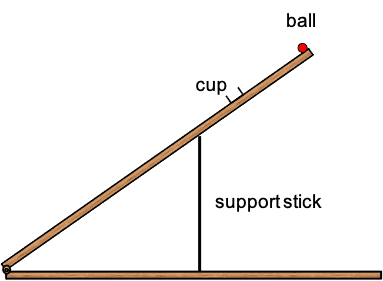# Rotation and torque about an accelerating point

• chris25
This demonstration works 100% of the time after the initial time investment of screwing the cup at the right spot (##L\cos\theta## from the hinge - small adjustments may be necessary).

#### chris25

Homework Statement
A uniform stick of mass M and length L is suspended horizontally with
end B on the edge of a table, while end A is held by hand. Point A is suddenly released. Right after release, find the vertical force at B, as well as the downward
acceleration of point A. You should find a result greater than g. Explain how this can be possible,
given that gravity is the only downward external force in the problem.
Relevant Equations
Question 6.14 from Kleppner and Kolenkow.
τ = dL/dt
A solution was provided:

We take torques about point B. Note that τ = MgL/2 = Iα so α = (3g)/2L. Everything from here is straightforward.

I don't understand why in this step, you can take torque about B without accounting for a fictitious force due to the acceleration of the Rod.

Thanks for any help.

chris25 said:
I don't understand why in this step, you can take torque about B without accounting for a fictitious force due to the acceleration of the Rod.
Welcome to PF!

The analysis of the solution is from the point of view of the inertial frame of the earth. So, in this frame there are no fictitious forces.

•PeroK and Lnewqban
TSny said:
Welcome to PF!

The analysis of the solution is from the point of view of the inertial frame of the earth. So, in this frame there are no fictitious forces.
Hi how is the reference frame inertial? Isn't the rod (and the point B in contact with the table) accelerating?

Welcome, @chris25 !
It seems to me that the “as well as the downward acceleration of point A” refers to the vertical acceleration of end A rather than the angular acceleration of the rod (α).

chris25 said:
Hi how is the reference frame inertial? Isn't the rod (and the point B in contact with the table) accelerating?
The rod's center of mass accelerates with respect to the earth and the rod also rotates relative to the earth. The frame of reference is the earth frame. The frame of reference is not a frame moving with the rod. When the solution finds the angular acceleration ##\alpha##, this is the angular acceleration of the rod with respect to the earth.

•PeroK, nasu and Lnewqban
chris25 said:
Isn't the rod (and the point B in contact with the table) accelerating?
Point B of the rod does not accelerate relative to the earth just after point A is released. Point B of the rod remains at rest relative to the earth just after the rod is released at point A. So, taking torques about point B of the rod is equivalent to taking torques about a point that is fixed relative to the earth.

•PeroK
Here is a nice demonstration of this idea. Two planks are hinged at one end, opened at about 35° and held in place by a support stick. The tilted plank has a small ball at its tip prevented from rolling by a dimple in the wood. A small metal cup or can, is screwed in place at an appropriate distance from the plank's tip.
Question: How can you put the ball in the cup without touching it?
Answer: Yank the supporting stick away.
How does it work? The tip's acceleration has magnitude greater than ##g## which means it separates from the ball fall. The ball falls freely in a straight line towards the point directly below it. The cup describes a circular arc and stops in time for the ball to drop in it.This demonstration works 100% of the time after the initial time investment of screwing the cup at the right spot (##L\cos\theta## from the hinge - small adjustments may be necessary). I highly recommend it and the students are impressed. It is a good idea to put some lossy material (silly putty works best) inside the cup to prevent the ball from bouncing right out.

••TSny, nasu, PeroK and 1 other person